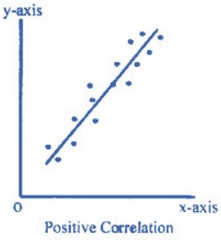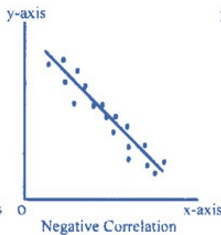Correlations

Description, AO1 Correlational Analysis:

A correlational analysis aims to assess whether there is a relationship between two variables, and the strength of that relationship.

The difference between correlational analysis and experiments is that two variables are measured (two DVs – known as co-variables). Correlational analysis requires quantitative data (in the form of numbers). For example, it could be used to measure the relationship between revision and exam.

Types of correlation

(1) Positive: A positive correlation refers to a relationship where the variables move in the same direction. E.g. an increase in one variable is accompanied by an increase in another variable.

For example, an increase in revision is accompanied by an increase in exam performance. Note that this also means that a decrease in revision is accompanied by a decrease in exam performance.

(2) Negative: Negative: A negative correlation refers to a relationship where the variables move in opposite directions. E.g. an increase in one variable is accompanied by a decrease in another variable.

For example, an increase in visits to the pub is accompanied by a decrease in exam performance.

Correlation Co-efficient

This is a number that tells us the strength and direction of the relationship between two variables. The correlation co-efficient varies between –1 and +1. On this scale -1 represents a perfect negative correlation, +1 represents a perfect positive correlation and 0 represents no correlation.Writing a Correlational Hypothesis Writing a hypothesis for a correlational analysis is very different to writing a hypothesis for a laboratory or field experiment. When writing a correlational hypothesis it is important that you state what you predict the relationship between the co-variables to be (e.g. there will be a significant positive correlation, negative correlation or, there will be no significant relationship).

For example,

(1) There will be a significant positive correlation between the amount of revision completed and the score obtained in an example, the more hours revision completed, the higher the score in an exam.

(2) There will be a significant negative correlation between the amount of alcohol consumed and an individuals reaction times, the more units of alcohol consumed the slower reaction times in seconds will be.

Evaluation, AO3 of Correlational Analysis:

Strengths:

(1) POINT: A strength of using a correlational analysis is that it allows a researcher to view the strength of a relationship between 2 variables. This means that a correlational analysis is an effective way of measuring the strength of relationships between two variables. EXAMPLE: For example, a correlation co-efficient of 0.8 indicates a strong positive relationship between two variables whereas a co-efficient of 0.3 indicates a relatively weak positive relationship. EVALUATION: This is positive because it enables the researcher to compare and contrast results easily and gain a better understanding of the relationship between different variables.

(2) POINT: A further strength of a correlational analysis is that it can prompt further research to be conducted. This means that correlational analysis can identify relationships between variables which may open up new lines of research. EXAMPLE: For example, further experimental research may indicate the likelihood of the relationship being causal or not. EVALUATION: This is positive because further research helps to expand our understanding of the world.

Weaknesses:

(1) POINT: A weakness of a correlational analysis is that is doesn’t allow a researcher to establish causation. This means that it is not possible to establish a cause and effect relationship between the variables in a correlation. EXAMPLE: For example, just because the healthier people’s diets are the better their memories are does not mean that a good diet causes the better memory, there is simply a link. EVALUATION: This is problematic as it limits the extent to which the researcher can firm draw conclusions from the research.

(2) POINT: A further weakness of conducting a correlational analysis is that the presence of a third variable cannot be ruled out. EXAMPLE: This means that we cannot be sure that the relationship that exists is definitely due to the two variables being studied and not due to something else (i.e. a third variable that is not being measured). For example, from studying the link between stress and illness we assume that they are linked, however, it may be that stress reduces the amount of sleep that a person is able to have and it is this that increases illness (sleep being the third variable). EVALUATION: This is problematic because it means correlations might be misleading.